# Goodbye Overall Harm, hello Specific Durability! (BIG IDEA = MUST READ)

Status
Not open for further replies.

#### PieFlinger

I've computed an alternative formula to the Overall Harm formula, which I call Specific Durability. Before I begin, a warning...

MATH WARNING: THERE IS MATH HERE

The difference is that while overall harm computes, well, the harm caused by a given damage constant, this formula calculates the minimum damage constant required to OHKO a pokemon with the given stats.

It is derived as follows:

Code:
``````(All variables are the same as in [URL="http://www.smogon.com/dp/articles/maximizing_defenses#overall"]Overall Harm.[/URL])
Damage = K/D + 2 + K/S + 2
Damage = K/D + K/S + 4``````
Now, as an OHKO occurs when Damage is greater than or equal to HP,
we can find the minimum damage constant required to OHKO:
Code:
``````H - (Damage) = 0
H = (Damage)
H = K/D + K/S + 4``````
(Now, we isolate K)
Code:
``````H - 4 = K(D + S)/(DS)
[I]K = (DS(H - 4))/(D + S)[/I]``````
Ta-Da! Overall durability! The higher K is, the more durable the pokemon, i.e. the more power it takes to KO it. Your goal: maximize K.

So, in the formula, K will equal the minimum attack constant of a physical attack followed by a special attack such that the combined damage from both attacks KOs the opponent.

Why, you may ask, is this formula better than Overall Harm?

I think it simplifies things. Rather than fiddling about with an extra variable, your Damage Constant, this formula eliminates unnecessary scalars and focuses on one thing: how much damage your pokemon can take (as opposed to how much a given attack will f*** your pokemon up).

Now, it's not too difficult from here to use the optimization and limitation formulas one would use to calculate the ideal EV spread.

What we'll do is make a formula for each stat which we'll plug in whenever we see a D, S, or H.

The formula is as follows:
Code:
``````E = Total EVs you're alloting to defenses

Eh, Es, Ed = HP, Sp.D, Def EVs, respectively

Sh, Ss, Sd = What each stat would be at your target level with 0 EVs

Eh + Es + Ed = E      (A limiter to make sure we don't exceed
the allotted Durability EVs)

L = 4*(100/Level)     (The reciprocal of the scalar of the rate
at which EVs add to each stat.
If you don't get it now, you will later. Basically,
Lv.50 makes it 8 and Lv.100 makes it 4.)

Then, we get:

H = Sh + Eh/L
S = Ss + Es/L
D = Sd + Ed/L

Now, we plug these expressions into
the Specific Durability formula, and get...

K = [U](Sd + Ed/L)(Ss + Es/L)(Sh + Eh/L - 4)[/U]
(Sd + Ed/L) + (Ss + Es/L)

Then, if you wanted to get all algebraic-y,
calculus-y about it, you might do this:

Let
E = (Total EVs available for distribution)/L
z = K
x = Ed/L
y = Es/L
Eh/L = E - x - y
x + y <=E          ("<=" means "less than or equal to")
x >= 0
y >= 0
(These last three because the subscripts get confusing)
H = Sh
D = Sd
S = Ss

As such,

z(x,y) = [U](x + D)(y + S)(H + E - x - y - 4)[/U]
(x + D) + (y + S)``````
That, if you plug it into a 3D grapher (try the TI-89, TI-84 (with a 3rd-party addon), or the Grapher program on Mac OSX 10.4 and later), it will render a 3D surface. Then, all you have to do is find the highest point within the given constraints.

Here's a simplified example of what the surface might look like:(And the optimum config would be the peak of that Math Mountain)

I'm sure there's a way to precisely find the optimum configuration without guess-and-check-ing, but it is beyond my Calculus skillz to figure it out. Or, alternatively, the different D, S, and H values seem to only transform the function, not change its symmetrical shape. That could be used to easily calculate with a VERY simple formula. Anyway, I must sleep, so that's all for now.

I really hope this is useful to the world of pokemanz, and I'd love it if you guys could give feedback/suggestions/further calculations regarding this new formula.

In the meantime, I shall snooze. Good luck.

#### Larvitar Star

this formula calculates the minimum damage constant required to OHKO a pokemon with the given stats
The only problem I can see with this is that it doesn't take stealth rock/other hazards into account. So if you are trying to give Tyranitar for instance the minimum SpA EV investment to OHKO dragonite, you could be wasting EV's if your team revolves around having Stealth Rock in play.

I like the idea though, amazing first post :)

#### david stone

##### Fast-moving, smart, sexy and alarming.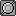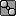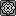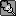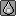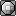Stealth Rock is easy to take into account. Just pretend HP = HP - SR damage

#### Aminar

Note to self, see if my DS can run a TI-89.

This is really really cool. Now if we could get a list of common damage numbers to run by it compiled. Maybe a benchmark of requiring a 2hko from a +1 outrage from Salamence, surviving a CB Head Smash from Aggron, etc. A program to check stuff could pretty easily be made to test this, but it's beyond my programming skills.

#### Zog

Nice idea. Would be useful in putting together defensive EVs, seeing as I've always just put 252 in hp due to it being useful on both ends.

#### PieFlinger

My apologies, noob3, I didn't mean to offend, I was just trying to ward off any obnoxious 8th graders who think they're too kool 4 skool. (And further apologies if your avatar is a self-portrait...) If you have trouble with math and are decent at competitive pokemon, maybe it would help you to think of math in terms of Pokemon? I dunno.

And anyone who wants to program/script this, be my guest. MatLab and/or Common Lisp might prove helpful to anyone undertaking such an endeavor.

Aminar: I doubt your DS can run TI-89 because the TI-89 is a calculator, not a program, but you easily get an emulator for your PC online. Or just obtain MatLab... If you want to go even further, you could scale the Def and SpD in accordance to the proportion and power of each move common in a given metagame.

Finally, if there are any math/statistics majors or minors or other mathletes with experience beyond year-one Calculus, your help would be awesome.
Because I can't do any more with the optimization beyond guess-and-check-ing: I actually tried to derive the simplified formula (E=100, S=H=D=0) in terms of x with y as an arbitrary constant, and gave up here:

Code:
``````[U]dz[/U] = (x^3) - (y^2)(x-E) - x - y - 4
dx``````
I think this is correct, but I have no idea where to go from here. Maybe computing the transformation resulting from changes in E, S, D, and H would be more fruitful...

Anyway, CALLING ALL MATHLETES! Your help is required!

#### Aminar

My DS runs a TI-83's stuff just fine, I'm not sure on the TI-89 though, may need more power. Homebrew is a wonderful thing.

#### Stylish Interval

I love the fact that you can't make a joke without insulting a bunch of lurkers :P

For quick stuff, I'd rather use the Smogon calculator's reverse calcs but I like that this is your first post and you're already well off (aside from with people who were stupid enough to take offense to a joke from someone they didn't know). I've got to say it looks interesting and I will be testing this out and seeing how it does and help you if I can. Good work *thumbs up*

#### Cathy

##### Banned deucer.
There really isn't anything to discuss here. The original post introduces a lot of notation, but doesn't give a single example of how this notation can be used to solve a pokemon problem, and the condescending tone and irrelevant 3D graph don't add anything.

Status
Not open for further replies.